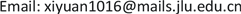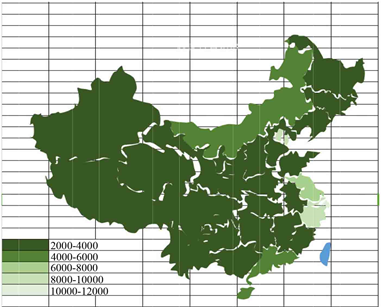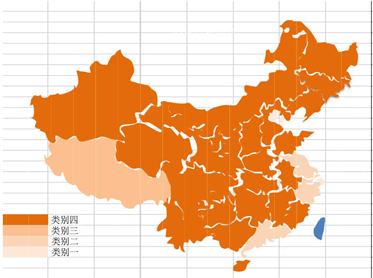﻿ “低保标准”的数学模型建立 The Establishment of Mathematical Model of “Subsistence Allowance Standard”

Statistics and Application
Vol. 08  No. 02 ( 2019 ), Article ID: 29722 , 17 pages
10.12677/SA.2019.82035

The Establishment of Mathematical Model of “Subsistence Allowance Standard”

Yuan Xi, Daihui Yu, Huiting Guo

Jilin University, Changchun JilinReceived: Mar. 25th, 2019; accepted: Apr. 9th, 2019; published: Apr. 16th, 2019ABSTRACT

In order to establish the mathematical model of the standard, we put forward the following four questions. Question 1: Select the main index to calculate the “subsistence allowance standard”. Question 2: For a certain region, according to the data obtained, the “subsistence allowance standard” of the region is given. Question 3: Analyze the correlation between the existing “subsistence allowance standards” in different regions (provinces, counties and cities). Question 4: Using the result of question 3, this paper gives a multivariate mathematical model and verifies the adaptability of the model.

Keywords:Uniform Matrix Method, Component Analysis, Regression Analysis, Clustering Analysis

“低保标准”的数学模型建立Copyright © 2019 by authors and Hans Publishers Inc.

This work is licensed under the Creative Commons Attribution International License (CC BY).

http://creativecommons.org/licenses/by/4.0/1. 问题重述

1.1. 问题背景

1.2. 问题提出

(1) 挑选主要计算“低保标准”的指标；

(2) 就某一地区，给出该地区“低保标准”，并给出模型分析与解释；

(3) 分析现行各地“低保标准”的相关性；

(4) 利用(3)分析的结果，给出多元数学模型，并给出模型适应性验证。

2. 模型假设

1) 假设不考虑自然灾害、金融危机等不可抗因素；

2) 假设所查阅的数据不存在误差。

3. 符号说明

${C}_{i}$ ：低保标准的六个指标；

${y}_{t}$ ：第t年的年低保标准的实际值；

${\stackrel{^}{y}}_{t}$ ：第t年的年低保标准的预测值；

${x}_{1t}$ ：第t年居民人均消费支出；

${x}_{2t}$ ：第t年该地区财政收入；

${\alpha }_{0}$ ：参数；

${\beta }_{i}$ ：参数；

$y$ ：年低保标准的实际值；

$\stackrel{^}{y}$ ：年低保标准的预测值；

${x}_{i}$ ：影响低保标准的六个变量。

4. 模型的建立与求解

4.1. 问题一的分析与建模

4.1.1. 模型一的建立及求解

${C}_{1},{C}_{2},{C}_{3},{C}_{4},{C}_{5},{C}_{6}$ 依次表示：地区生产总值、人均消费支出、一般公共预算社会保障和就业支出、居民消费价格指数、商品零售价格指数、政府财政收入。所构造的成对比较矩阵如下：

$A=\left[\begin{array}{cccccc}1& 1/5& 1/2& 2& 2& 1/3\\ 5& 1& 3& 6& 6& 2\\ 2& 1/3& 1& 3& 3& 1/2\\ 1/2& 1/6& 1/3& 1& 1& 1/4\\ 1/2& 1/6& 1/3& 1& 1& 1/4\\ 3& 1/2& 2& 4& 4& 1\end{array}\right]$

4.1.2. 模型一的评价与改进

4.1.3. 模型二的建立及求解Table 1. Urban subsistence allowance standards and indicators

Table 2. Rural subsistence allowance standards and indicators

4.2. 问题二的分析与建模

4.2.1. 城市“低保标准”数学模型建立与求解Table 7. Urban subsistence allowance standard of Jilin province, per capita consumption expenditure of urban residents and fiscal revenue data of this region

${y}_{t}={\beta }_{0}+{\beta }_{1}{x}_{1t}+{\beta }_{2}{x}_{2t}+{\epsilon }_{t}$ (1)

${\stackrel{^}{y}}_{t}=-2.1448+0.4445{x}_{1t}-1.0692{x}_{2t}$ (2)

${y}_{t}={\beta }_{0}+{\beta }_{1}{x}_{1t}+{\beta }_{2}{x}_{2t}+{\epsilon }_{t},{\epsilon }_{t}=\rho {\epsilon }_{t-1}+{u}_{t}$ (3)

$\rho$ 是自相关系数， $|\rho |\le 1$${u}_{t}$ 相互独立且服从均值为零的正态分布， $t=1,2,\cdots ,n.$

$DW=\frac{{\sum }_{t=2}^{n}{\left({e}_{t}-{e}_{t-1}\right)}^{2}}{{\sum }_{t=2}^{n}{e}_{t}^{2}}$ (4)

$DW=2\left(1-\frac{{\sum }_{t=2}^{n}{e}_{t}{e}_{t-1}}{{\sum }_{t=2}^{n}{e}_{t}^{2}}\right)$ (5)

$\frac{{\sum }_{t=2}^{n}{e}_{t}{e}_{t-1}}{{\sum }_{t=2}^{n}{e}_{t}^{2}}$ 是自相关系数 $\rho$ 的估计值 $\stackrel{^}{\rho }$ ，所以：

$DW\approx 2\left(1-\stackrel{^}{\rho }\right)$ (6)

$\stackrel{^}{\rho }=1-\frac{DW}{2}=0.8975$ (7)

${y}_{t}^{*}={y}_{t}-\rho {y}_{t},{x}_{it}^{*}={x}_{it}-\rho {x}_{i,t-1},t=1,2$ (8)

${y}_{t}^{*}={\beta }_{0}^{*}+{\beta }_{1}^{*}{x}_{1t}^{*}+{\beta }_{2}^{*}{x}_{2t}^{*}+{u}_{t},{\beta }_{0}^{*}={\beta }_{0}\left(1-\rho \right)$ (9)

${\stackrel{^}{y}}_{t}=-0.0102+0.8975{y}_{t-1}++0.3932{x}_{1t}-0.3528{x}_{1,t-1}-1.5132{x}_{2t}+1.3581{x}_{2,t-1}$ (10)Table 12. Calculation values of model (10) and model (2)

4.2.2. 农村问题的建模与求解

${y}_{t}={\beta }_{0}+{\beta }_{1}{x}_{1t}+{\beta }_{2}{x}_{2t}+{\epsilon }_{t}$ (11)

${\stackrel{^}{y}}_{t}=-0.4637+0.3667{x}_{1t}-0.1466{x}_{2t}$ (12)Table 13. The annual subsistence allowance standard of rural areas in Jilin province, the per capita consumption expenditure of rural residents and the fiscal revenue data of this region

${y}_{t}={\beta }_{0}+{\beta }_{1}{x}_{1t}++{\beta }_{2}{x}_{2t}+{\epsilon }_{t},{\epsilon }_{t}=\rho {\epsilon }_{t-1}+{u}_{t}$ (13)

$\rho$ 是自相关系数， $|\rho |\le 1$${u}_{t}$ 相互独立且服从均值为零的正态分布， $t=1,2,\cdots ,n$

$DW=\frac{{\sum }_{t=2}^{n}{\left({e}_{t}-{e}_{t-1}\right)}^{2}}{{\sum }_{t=2}^{n}{e}_{t}^{2}}$ (14)

$DW=2\left(1-\frac{{\sum }_{t=2}^{n}{e}_{t}{e}_{t-1}}{{\sum }_{t=2}^{n}{e}_{t}^{2}}\right)$ (15)

$\frac{{\sum }_{t=2}^{n}{e}_{t}{e}_{t-1}}{{\sum }_{t=2}^{n}{e}_{t}^{2}}$ 是自相关系数 $\rho$ 的估计值 $\stackrel{^}{\rho }$ ，因此：

$DW\approx 2\left(1-\stackrel{^}{\rho }\right)$ (16)

4.3. 问题三的分析与建模

4.3.1. 问题的建模与求解

4.3.2. 结果分析

1) 类别一Figure 1. Distribution of national urban subsistence allowance standardFigure 2. Distribution of national rural subsistence allowance standardFigure 3. Distribution of national subsistence allowance standardTable 17. Classification of the country's 31 provinces

2) 类别二

3) 类别三

4) 类别四

4.4. 问题四的分析与建模

4.4.1. 模型的建立与求解

${x}_{1}=\left\{\begin{array}{l}9.56,\text{ }类别一;\\ 6.64,\text{ }类别二;\\ 5.12,\text{ }类别三;\\ 4.31,\text{ }类别四.\end{array}\text{ }{x}_{2}=\left\{\begin{array}{l}1,城市;\\ 0,农村.\end{array}\text{ }{x}_{4}=\left\{\begin{array}{l}0,城市;\\ 1,农村.\end{array}$

${x}_{3}$ 表示城市居民人均消费， ${x}_{5}$ 表示农村居民人均消费， ${x}_{6}$ 表示该地区财政收入。

$y={\alpha }_{0}+{\alpha }_{1}{x}_{1}+{\alpha }_{2}{x}_{2}{x}_{3}+{\alpha }_{3}{x}_{4}{x}_{5}+{\alpha }_{4}{x}_{6}$ (17)

$\stackrel{^}{y}=-0.9474+0.6373{x}_{1}+0.1710{x}_{2}{x}_{3}+0.1994{x}_{4}{x}_{5}-0.0615{x}_{6}$ (18)

Table 21. The true, predicted and residual values of the subsistence allowance standard in 2016

4.4.2. 模型的适应性验证

Table 22. The true, predicted and residual values of the subsistence allowance standard in 2015

5. 模型评价

1) 本文建立的模型与实际生活紧密相连，对各省低保标准和主要指标进行建模，其结果与实际相符。对民政部门，统计部门都具有很高的参考价值。

2) 模型建立的模型依据的数据来自《中国统计年鉴》以及中国民政部所给出的数据，使得模型的可信度较高。

3) 模型具有一定创新性和灵活性，对各省的情况都符合。

1) 对省份的分类不够细致，在实际中应用会有一定误差。

2) 成对比较矩阵，可以实现权重计算，但由于其标度的选择具有较大的主观性，且无法体现出城市与农村“低保”指标的差异性，因此所得出的结果不够具有说服力。

3) 问题二中在针对吉林省研究该地区的低保标准时，由于官网上找不到大量年份的低保标准，所以在研究此问题时的数据量不够大，无法深层次地再研究2016年以前低保标准的适应性。

The Establishment of Mathematical Model of “Subsistence Allowance Standard”[J]. 统计学与应用, 2019, 08(02): 303-319. https://doi.org/10.12677/SA.2019.82035

1. 1. 李春根, 夏俊. 中国城市最低生活保障标准: 变化轨迹与现实考量[J]. 中国行政管理, 2014(354): 90-94.

2. 2. 姜启源, 谢金星, 叶俊. 数学模型[M]. 第四版. 北京: 高等教育出版社, 2011: 346-352.

3. 3. 中华人民共和国国家统计局. 中国统计年鉴[R]. http://www.stats.gov.cn/tjsj/ndsj/, 2018-04-29.

4. 4. 中华人民共和国民政部. 民政数据统计季报[R]. http://www.mca.gov.cn/article/sj/tjjb/qgsj/, 2018-04-29.